### Home > MC1 > Chapter 9 > Lesson 9.1.1 > Problem9-8

9-8.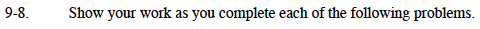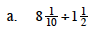This problem is very similar to 9-7. Refer to that problem if you are having difficulty starting this problem.

$5\frac{2}{5}$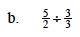Since three-thirds is equal to one, you are actually dividing five-halves by one.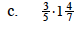It may be best to change the mixed number into a fraction greater than one before you multiply.

$\frac{33}{35}$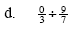Look carefully at this problem.
Do you notice anything interesting?

Zero-thirds is equal to zero.
What happens when you divide zero by any number?